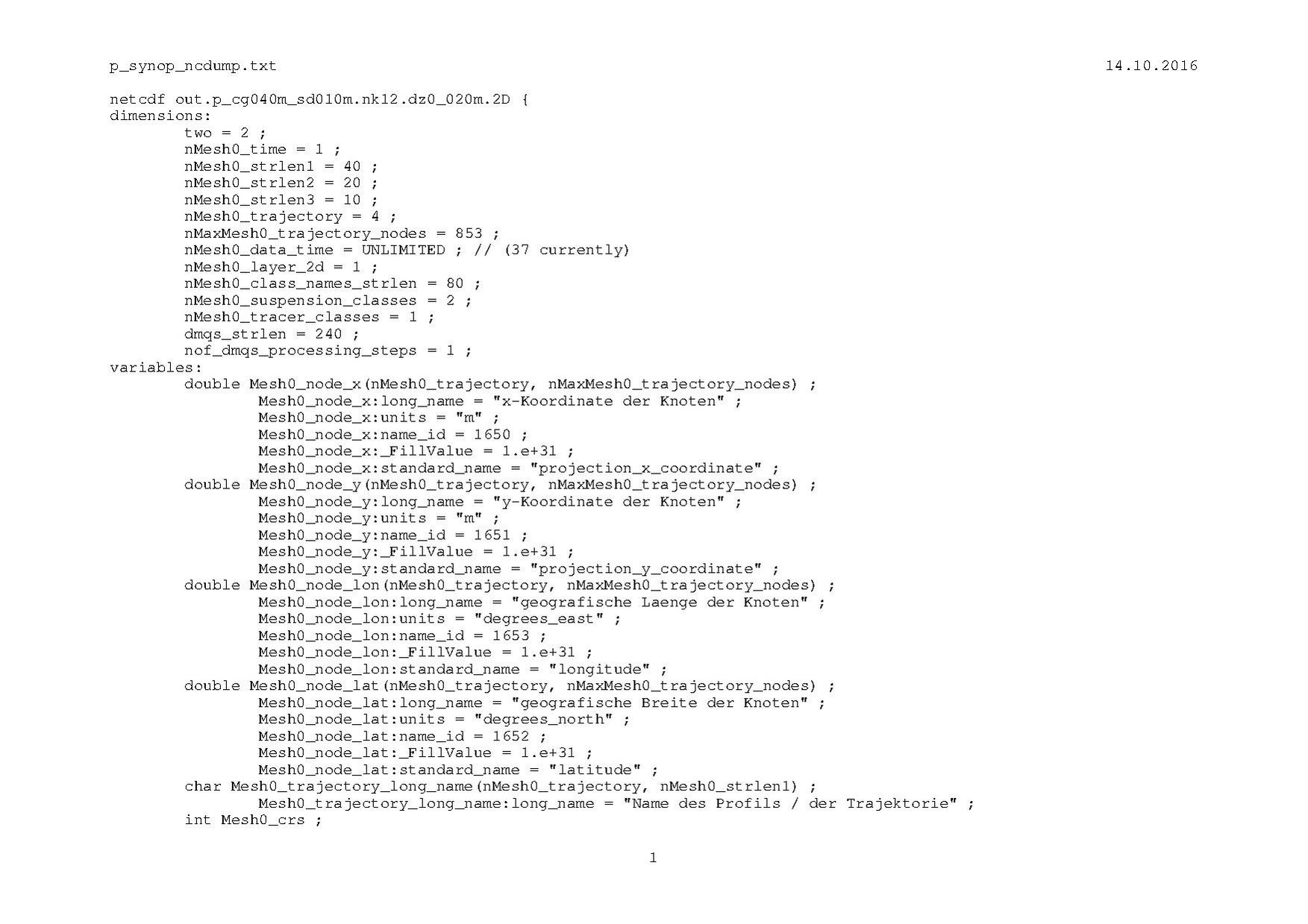# Introduction

The location of different longitudinal as well as cross-sectional profiles is described.

# Version Discrete Sampling Geometry trajectoryProfile

Data for multiple locations along one or several profiles (trajectories) are stored in a netCDF file in accordance with the concept of a Discrete Sampling Geometry with featureType=trajectoryProfile. An example (output from NCDUMP) for geometry as well as data is shown in.

Short list and description of some relevant variables for profiles:

• Mesh0_node_x(nMesh0_trajectory,nMaxMesh0_trajectory_nodes): x-coordinate;
• Mesh0_node_y(nMesh0_trajectory,nMaxMesh0_trajectory_nodes): y-coordinate;
• Mesh0_node_lon(nMesh0_trajectory,nMaxMesh0_trajectory_nodes): geographic longitude;
• Mesh0_node_lat(nMesh0_trajectory,nMaxMesh0_trajectory_nodes): geographic latitude;
• Mesh0_trajectory_node_distance(nMesh0_trajectory,nMaxMesh0_trajectory_nodes): profile meter;
• Mesh0_trajectory_long_name(nMesh0_trajectory,nMesh0_strlen1): long name;
• Mesh0_trajectory_code_name(nMesh0_trajectory,nMesh0_strlen2): short name;
• Mesh0_trajectory_short_name(nMesh0_trajectory,nMesh0_strlen3): shorthand name;
• Mesh0_trajectory_id(nMesh0_trajectory): identification number, e. g. with cf_role matching respective featureType;
• Mesh0_crs: scalar container variable with informations (contents of attributes) about the coordinate transformation used (relationship between local and geographical coordinates).

Remarks:

1. All two-dimensional coordinate variables may contain invalid data (_FillValue). This will always be the case if individual profiles (1 to nMesh0_trajectory) consist out of different number of locations (1 to nMaxMesh0_trajectory_nodes).
2. Variable Mesh0_trajectory_node_distance can be used as an auxiliary coordinate variable in geophysical variables. With this variable displaying data in dependence on profile meter is largely facilitated.

# Version DATACONVERT

The basic concept follows the ideas described in 1D network topolgy which is part of the UGRID Conventions. But use of object profile is beyond what is covered by the UGRID conventions. All deviations from the UGRID conventions are highlighted in darkorange color.

## Dimensions

1. nMesh1_node : number of nodes
2. nMesh1_edge : number of edges
3. nMesh1_prof : number of profiles (longitudinal and cross-section)
4. nMaxMesh1_prof_edges : maximum number of edges along a single profile
5. nMaxMesh1_prof_nodes : maximum number of nodes along a single profile
6. Two : constant ( = 2 ).

## Local coordinates

### Nodes

double Mesh1_node_x(nMesh1_node) ;

Mesh1_node_x:long_name = "x-coordinate of nodes ;
Mesh1_node_x:units = "m" ;
Mesh1_node_x:name_id = 1650 ;
Mesh1_node_x:standard_name = "projection_x_coordinate" ;

double Mesh1_node_y(nMesh1_node) ;

Mesh1_node_y:long_name = "y-coordinate of nodes" ;
Mesh1_node_y:units = "m" ;
Mesh1_node_y:name_id = 1651 ;
Mesh1_node_y:standard_name = "projection_y_coordinate" ;

Comment:

1. "name_id" corresponds to the so-called PHYDEF-Code of variables which is used internally at BAW.

### Edges

double Mesh1_edge_x(nMesh1_edge) ;

Mesh1_edge_x:long_name = "x-coordinate of edges (center)" ;
Mesh1_edge_x:units = "m" ;
Mesh1_edge_x:name_id = 1650 ;
Mesh1_edge_x:bounds = "Mesh1_edge_x_bnd" ;
Mesh1_edge_x:standard_name = "projection_x_coordinate" ;

double Mesh1_edge_y(nMesh1_edge) ;

Mesh1_edge_y:long_name = "y-coordinate of edges (center)" ;
Mesh1_edge_y:units = "m" ;
Mesh1_edge_y:name_id = 1651 ;
Mesh1_edge_y:bounds = "Mesh1_edge_y_bnd" ;
Mesh1_edge_y:standard_name = "projection_y_coordinate" ;

Comment:

1. "name_id" corresponds to the so-called PHYDEF-Code of variables which is used internally at BAW.

### (optional) Bounds for edges

double Mesh1_edge_x_bnd(nMesh1_edge,Two) ; \\ no metadata required

double Mesh1_edge_y_bnd(nMesh1_edge,Two) ; \\ no metadata required

## Geographic coordinates

### Nodes

double Mesh1_node_lon(nMesh1_node) ;

Mesh1_node_lon:long_name = "geographic longitude of nodes" ;
Mesh1_node_lon:units = "degrees_east" ;
Mesh1_node_lon:name_id = 1653 ;
Mesh1_node_lon:standard_name = "longitude" ;

double Mesh1_node_lat(nMesh1_node) ;

Mesh1_node_lat:long_name = "geographic latitude of nodes" ;
Mesh1_node_lat:units = "degrees_north" ;
Mesh1_node_lat:name_id = 1652 ;
Mesh1_node_lat:standard_name = "latitude" ;

Comment:

1. "name_id" corresponds to the so-called PHYDEF-Code of variables which is used internally at BAW.

### Edges

double Mesh1_edge_lon(nMesh1_edge) ;

Mesh1_edge_lon:long_name = "geographic longitude of edges (center)" ;
Mesh1_edge_lon:units = "degrees_east" ;
Mesh1_edge_lon:name_id = 1653 ;
Mesh1_edge_lon:bounds = "Mesh1_edge_lon_bnd" ;
Mesh1_edge_lon:standard_name = "longitude" ;

double Mesh1_edge_lat(nMesh1_edge) ;

Mesh1_edge_lat:long_name = "geographic latitude of edges (center)" ;
Mesh1_edge_lat:units = "degrees_north" ;
Mesh1_edge_lat:name_id = 1652 ;
Mesh1_edge_lat:bounds = "Mesh1_edge_lat_bnd" ;
Mesh1_edge_lat:standard_name = "latitude" ;

Comment:

1. "name_id" corresponds to the so-called PHYDEF-Code of variables which is used internally at BAW.

### (optional) Bounds for edges

double Mesh1_edge_lon_bnd(nMesh1_edge,Two) ; \\ no metadata required

double Mesh1_edge_lat_bnd(nMesh1_edge,Two) ; \\ no metadata required

## Connectivities

### Edge-node connectivity

int Mesh1_edge_nodes(nMesh1_edge, two) ;

Mesh1_edge_nodes:long_name = "edge-node connectivity of edges, start and end point" ;
Mesh1_edge_nodes:cf_role = "edge_node_connectivity" ;
Mesh1_edge_nodes:start_index = 0 ;

### Names of profiles

char Mesh1_prof_long_name(nMesh1_prof, nMesh1_strlen1) ;

Mesh1_prof_long_name:long_name = "name of profile" ;

Comment:

1. Can be used as label coordinate variable to access individual profiles.

### Profile-edge connectivity

int Mesh1_prof_edges(nMesh1_prof, nMaxMesh1_prof_edges) ;

Mesh1_prof_edges:long_name = "profile-edge connectivity, sorted from start to end of profile" ;
Mesh1_prof_edges:cf_role = "prof_edge_connectivity" ;
Mesh1_prof_edges:_FillValue = -999 ;
Mesh1_prof_edges:start_index = 0 ;

Comment:

1. Can be used to describe several profiles.
2. Individual edges may belong to more than one profile.

### Profile-node connectivity

int Mesh1_prof_nodes(nMesh1_prof, nMaxMesh1_prof_nodes) ;

Mesh1_prof_nodes:long_name = "profile-node connectivity, sorted from start to end of profile" ;
Mesh1_prof_nodes:cf_role = "prof_node_connectivity" ;
Mesh1_prof_nodes:_FillValue = -999 ;
Mesh1_prof_nodes:start_index = 0 ;

Comment:

1. Can be used to describe several profiles.

## Topology

int Mesh1 ;

Mesh1:long_name = "several 1D profiles, no sub-grid" ;
Mesh1:topology_dimension = 1 ;
Mesh1:cf_role = "mesh_topology" ;
Mesh1:node_coordinates = "Mesh1_node_x Mesh1_node_y Mesh1_node_lon Mesh1_node_lat" ;
Mesh1:edge_coordinates = "Mesh1_edge_x Mesh1_edge_y Mesh1_edge_lon Mesh1_edge_lat" ;
Mesh1:prof_coordinates = "Mesh1_prof_long_name" ;
Mesh1:prof_node_connectivity = "Mesh1_prof_nodes" ;
Mesh1:prof_edge_connectivity = "Mesh1_prof_edges" ;
Mesh1:edge_node_connectivity = "Mesh1_edge_nodes" ;

back to NetCDF